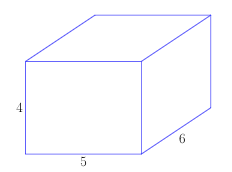# Surface Area of a Right Rectangular Prism

This post about finding the surface area of a right rectangular prism is part of a series of posts to help you prepare for the Advanced Algebra and Functions part of the Accuplacer test.

## Question

What is the surface area of the right rectangular prism shown? Its length is 6, its width is 5, and its height is 4.Right rectangular prism for the example

## Solution

### Sidebar: Surface Area of a Right Rectangular Prism

A right rectangular prism is the shape of a box with a rectangle for a bottom, with sides perpendicular to its bottom, and with a flat top parallel to its bottom. It’s your typical box.

A right rectangular prism’s surface area is the sum of the areas of all its faces: the top, the bottom, and the four sides. The area of each side is length times width.

This box has six sides, three pairs of congruent sides.

• Let’s start with the side that stares you in the face on the diagram. It measures 4 x 5, so its area is 20. There is an identical piece in the back with the same area; the area of the two together is 2 times 20 = 40.
• Now consider the sides on the left and right. (In the diagram, you can’t see the side on the left.) Each of those sides measures 6 x 4, so each one’s area is 24. The area of the two together is 2 times 24 = 48.
• The top has a length of 6 and a width of 5, so its area is 6 X 5 = 30. The bottom has the same area, so the area of the two together is 2 times 30 = 60.

Then the surface area of the whole prism is

And the answer is 148 square units.Right rectangular prism for formula

### In general

The formula for the surface area of a right rectangular prism — a box — is

where SA surface area and h, w, and l are as shown on the box on the right.

This question is similar to question number 3 in the sample questions for the Accuplacer Advanced Algebra and Functions test.

0 replies